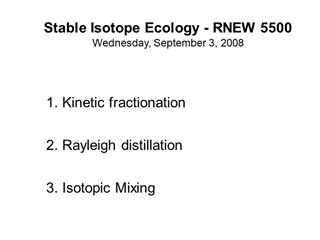# Stable Isotope Ecology - RNEW 5500 Wednesday, September 3, 2008, Kinetic fractionation Rayleigh distillation IsoMixing

0

#### Presentation Transcript

• 1.Stable Isotope Ecology - RNEW 5500 Wednesday, September 3, 2008 Kinetic fractionation Rayleigh distillation Isotopic Mixing
• 2.Non-equilibrium (kinetic) fractionation Forward reaction rate is accelerated relative to backward reaction and opportunity for backward mixing diminishes reactant product Change in temperature Product removal Most biological (enzyme) reactions
• 3.Unidirectional reactions (e.g., enzyme reactions) Ratio of reaction rate constants (k) determine fractionation where k1 and k2 are reaction rate constants for the light and heavy isotopes, respectively Non-equilibrium (kinetic) fractionation
• 4.Diffusive fractionation (a type of non-equilibrium or “kinetic” fractionation) During diffusion into a vacuum, a is the ratio of the velocities of the two isotopes, which is related to their masses:
• 5.Isotope fractionation and Rayleigh distillation (sometimes called Rayleigh fractionation)
• 6.Rayleigh fractionation/distillation Rayleigh fractionation occurs when a substrate mass is depleted during a physicochemical reaction and product is removed from system. The equation describing Rayleigh processes is: Rt = R0f (1-a) R and R0 are the ratios at t and at t=0 f is the fraction remaining at t a is the equilibrium fractionation factor Condensation example - condensate is fromed from a vapor mass and fraction remaining of original vapor declines
• 7.Rayleigh fractionation creates differences in d values between source and product masses (e.g., liquid and vapor) Rayleigh fractionation describes the process of ISOTOPE FRACTIONATION as a liquid pool evaporates (e.g., ephemeral pond) or as a vapor mass condenses (e.g., rainfall) Evaporation example - vapor is formed from the liquid and the fraction remaining of original liquid declines, as in an evaporating lake
• 8.As Rayleigh fractionation proceeds during evaporation, the d value of the accumulated vapor mass and the remaining water change. The pattern is dependent on whether you have a “CLOSED” or an “OPEN” system. The term “Rayleigh fractionation” is typically applied to the case of the OPEN system, not the closed-system (or “batch”) isotopic fractionation process. Evaporation example
• 9.In a CLOSED system, the vapor pool is in continuous contact with the liquid pool, and: dL = [adtot + 1000f(a-1)]/[a(1-f)+f] where f is residual water fraction, dtot is the d value of the total water, and a is the equilibrium fractionation between liquid and vapor. A = d18O of water in a CLOSED system B = d18O of vapor in a CLOSED system” A B Evaporation example
• 10.e e e As distillation proceeds in a CLOSED system the two pools remain in contact, thus the liquid and vapor d values always differ by e owing to equilibration based on the fractionation factor (a)
• 11.In an OPEN system, instantaneous vapor is in equilibrium with the water but this vapor fraction is removed soon after formation and accumulates (cumulative vapor) elsewhere: Rt = R0f (1-a) R and R0 are the ratios at t and at t=0, f is the fraction remaining at t and a is the equilibrium fractionation factor. In delta notation: dL = (1000 + d0)f(a-1) - 1000 A = remaining water in OPEN system (liquid) B = instantaneous vapor in OPEN system C = accumulated vapor fraction being removed from the OPEN system A B C Evaporation example
• 12.In an OPEN system, the remaining liquid (A) always differs from the instantaneous vapor (B) by the equilibrium enrichment value (e), but the accumulated vapor (C) becomes progressively more different from the remaining liquid.      A B C Evaporation example
• 13.For all systems, if distillation is complete, the accumulated vapor mass must have a d value equal to the initial water mass Evaporation example
• 14.Isotope mixing Linear mixing two isotope mixtures are related by: dsample = X dA + (1-X) dB IF the concentrations of the mixed phase are equal in each reservoir. (e.g., mixing waters with two different d18O or d2H values). This works because 16O ≈ 1 and H ≈ 1.0 (i.e., 16O >> 18O, H >> D). Concentration dependent mixing dsample = (CAmAdA + CB mBdB) / (CAmA + CBmB) This applies if the concentrations are different in the reservoirs such as mixing of sulfate pollution between two different waters.
• 15.Fractional mixing when concentrations differ